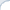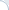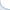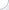The Hugging Face Accelerate library provides a convenient set of primitives for distributed training on multiple devices including GPUs, TPUs, and hybrid CPU-GPU systems (via Deepspeed). Despite convenience, there is one drawback: Only a synchronous SGD is supported. After gradients are computed (possibly in a series of accumulation steps), they are synchronized among devices and the model is updated. However, gradient synchronization is costly and it is particularly costly for consumer-grade GPUs, which are connected via PCI Express.

For example, if you have a 4-GPU server with a 16-lane PCI express v3, your synchronization capacity seems to be limited to 16 GB per second . Without fast GPU interconnect, gradient synchronization requires transferring of each model weights to CPU memory with subsequent transfers to three other GPUs. This would be 16 transfers in total. If PCI express is fully bidirectional (which seems to be the case), this can be done a bit more efficiently (with 12 transfers) . According to my back-of-the-envelope estimation gradient synchronization can take about the same time as training itself ! Thus, there will be little (if any) benefit of multi-GPU training.

Without further speculation, let us carry out an actual experiment (a simple end-to-end script to do so is available). I train a BERT large model for a QA task using two subsets of SQuAD v1 dataset (4K and 40K samples) using either one or four GPUs. Each experiment was repeated three times using different seeds. All results (timings and accuracy values) are provided.

In the multi-GPU setting, I use either a standard fully synchronous SGD or an SGD that synchronizes gradients every K batches. Note that the non-synchronous variant is hacky proof-of-concept (see the diff below), which likely does not synchronize all gradients (and it may be better to synchronize just model weights instead), but it still works pretty well.

For the fully synchronous SGD, each experiment is carried out using a varying number of gradient accumulation steps. If I understand the code of Accelerate correctly, the more accumulation steps we make, the less frequent is synchronization of gradients, so having more accumulation steps should permit more efficient training (but the effective batch size increases).

# of training
samples
Single-GPU Multi-GPU (four GPUs)
fully synchronous SGD
varying # of gradient accumulation steps
Multi-GPU (four GPUs)
k-batch synchronous SGD
varying # of gradient synchronization steps
1 2 4 8 16 1 2 4 8 16
4000 f1=79.3 f1=77.8 2.6x f1=74.7 2.7x f1=70.6 2.7x f1=54.8 2.9x f1=15.9 3.1x f1=77.4 2.6x f1=74.5 2.9x f1=71.9 3.3x f1=72.8 3.5x f1=74.2 3.6x
40000 f1=89.2 f1=88.6 2.4x f1=88.2 2.5x f1=87.5 2.6x f1=86.7 2.6x f1=84.4 2.6x f1=88.8 2.4x f1=87.2 2.8x f1=87.3 3.2x f1=87.4 3.4x f1=87.3 3.6x

The result table shows both the accuracy (F1-score) and the speed up with respect to a single-GPU training. First, we can see that using a small training set results in lower F1-scores (which is, of course, totally expected). Second, there is a difference between single-GPU training and fully-synchronous SGD, which is likely due to increase in the effective batch size (when all four GPUs are used). For the larger 40K training set the degradation is quite small. In any case, we use F1-score for the fully synchronous multi-GPU training as a reference point for the perfect accuracy score.

When we use the fully synchronous SGD, the increase of the number of gradient accumulation steps leads only to a modest speed up, which does not exceed 2.6x for the larger 40K set. At the same time, there is a 5% decrease in F1-score on the larger set and a catastrophic 3x reduction for the 4K set! I verified this dramatic loss cannot be easily fixed by changing the learning rate (at least I did not find good ones).

In contrast, for the non-synchronous SGD, there is a much smaller loss in F1-score when the synchronization interval increases. For the larger 40K training set, synchronizing one out of 16 batches leads to only 1.7% loss in F1-score. In that, the speed-up can be as high as 3.6x. Thus, our POC implementation of the non-synchronous SGD, which as I mentioned earlier is likely to be slightly deficient, is (nearly) always (often much) better than the current fully synchronous SGD implemented in Accelerator.

To reiterate, Accelerator supports only the synchronous SGD, which requires a costly synchronization for every batch. This is not an efficient setup for servers without a fast interconnect. A common "folklore" approach (sorry, I do not have a precise citation) is to relax this requirement and synchronize model weights (or accumulated gradients) every K>1 batches . This is the approach I implemented in FlexNeuART and BCAI ART. It would be great to see this approach implemented in Accelerator as well (or directly in Pytorch).

Notes:

 Interconnect information can be obtained via nvidia-smi -a.

 I think fewer than 12 bidirectional transfers would be impossible. Optimistically we can assume updated weights/gradients are already in the CPU memory, then each model weights/gradients need to be delivered to three other GPUs. In practice, 12 transfers are actually possible by moving data from one GPU's memory to CPU memory and immediately to another GPU's memory. After four such bi-directional transfers all data would be in the CPU memory. Thus, to finalize the synchronization process we would need only eight additional unidirectional (CPU-to-GPU) transfers.

 For a BERT large model (345M parameters) with half-precision gradients each gradient synchronization entails moving about 0.67 GB of data. As mentioned above, synchronization requires 12 bidirectional transfers for a total of 12 x 0.67 = 8GB of data. Thus, we can synchronize only twice per second. At the same time, when using a single GPU the training speed of BERT large on SQuAD QA data is three iteration/batches per second. Thus, gradient synchronization could take about the same time as training itself! My back-of-the-envelope calculations can be a bit off (due to some factors that I do not take into account), but they should be roughly in the ballpark.

 The parameter value K needs to be tuned. However, I find that its choice does not affect accuracy much unless K becomes too large. Thus it is safe to increase K until we achieve a speed-up close to the maximal possible one (e.g., 3.5x speed up with four GPUs). In my (admittedly limited) experience, this never led to noticeable loss in accuracy and sometimes it slightly improved results (apparently because non-synchronous SGD is a form of regularization).

A partial diff. between the original (fully-synchronous) and K-batch synchronous trainer (this is just a POC version, which is not fully correct):

@@ -760,6 +767,9 @@         num_training_steps=args.max_train_steps,     ) +    orig_model = model+    orig_optimizer = optimizer+     # Prepare everything with our accelerator.     model, optimizer, train_dataloader, eval_dataloader, lr_scheduler = accelerator.prepare(         model, optimizer, train_dataloader, eval_dataloader, lr_scheduler@@ -834,6 +845,7 @@      for epoch in range(starting_epoch, args.num_train_epochs):         model.train()+        orig_model.train()         if args.with_tracking:             total_loss = 0         for step, batch in enumerate(train_dataloader):@@ -842,17 +854,27 @@                 if resume_step is not None and step < resume_step:                     completed_steps += 1                     continue-            outputs = model(**batch)+            grad_sync = (step % args.no_sync_steps == 0) or (step == len(train_dataloader) - 1)+            if grad_sync:+                curr_model = model+                curr_optimizer = optimizer+            else:+                curr_model = orig_model+                curr_optimizer = orig_optimizer+            outputs = curr_model(**batch)             loss = outputs.loss             # We keep track of the loss at each epoch             if args.with_tracking:                 total_loss += loss.detach().float()             loss = loss / args.gradient_accumulation_steps-            accelerator.backward(loss)+            if grad_sync:+                accelerator.backward(loss)+            else:+                loss.backward()             if step % args.gradient_accumulation_steps == 0 or step == len(train_dataloader) - 1:-                optimizer.step()+                curr_optimizer.step()                 lr_scheduler.step()-                optimizer.zero_grad()+                curr_optimizer.zero_grad()                 progress_bar.update(1)                 completed_steps += 1 @@ -896,6 +918,7 @@     all_end_logits = []      model.eval()+    orig_model.eval()      for step, batch in enumerate(eval_dataloader):         with torch.no_grad():